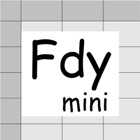• SEARCH TYPE

All iPhone applications categories

All iPhone games categories0 0

## Screenshots

Description

Faraday Calculator mini is a calculation sheet to solve problems of the Faraday's law of electrolysis. This Faraday Calculator mini is a subset of iPad Faraday Calculator software.
Faraday Calculator mini Lite is a Lite version of the Faraday Calculator mini software. This can operate the P button up to 2 times. When 10 minutes pass and the software is resumed, the P button can be used again.
This software solves the problem not as a black box but as a white box. You need to think the process of solving a problem. This makes your knowledge refresh. The black box solution is convenient, however it has a risk of losing your knowledge. This software has few such risks.

A typical problem is as follows.

(Example)
A factory is planning to produce aluminum by 1000kg a day.
How much current is needed?

//Step 0: Preparation//
Prepare chemical equations and atomic (molecular) weights.
This chemical equation needs to include electrons to determine z value.
They are as follows.
Al3+ + 3e- -> Al
Determine substance and z value. The z value is the number of electrons reacting to one substance particle.
The substance is Al and the z value is 3, since three electrons are reacting to one Al particle.
Atomic weight of Al = 26.98 [g/mol]

//Step 1: Definition//
Enter known data into the Fdy mini sheet.
Aw = 26.98, z = 3, W = 1000000, t = 24[h]

//Step 2: Calculation//
Data are not independent but mutually related by formulae.
Calculation is a conversion from unknown data to known data using the formulae.
The formulae are embedded in P and G buttons.
Touching out those buttons executes the formulae and changes unknown data to known data.
Touch out buttons as follows.
Touch out P_W button. Mol and charge data are changed to known data.

Set current unit to [A].
Touch out G_Ｉ(current) button. Current data is changed to known data.
It turns out that the needed current is 124173 A, i.e. 124 kA.

[Data label]
Aw [g/mol]: Atomic or molecular weight of substance.
z: number of electrons reacting to one substance particle.
W [g]: Substance weight.
Mol n [mol]: Substance mol quantity.
Q [C]: Charge
I [mA][A]: Current.
T (t) [s][m][h]: Time

[Buttons and data]
//P button and P data group//
P (put) button inputs data to which the P button is assigned.
P data group is a group of data to which the P buttons are assigned. The P data group has a special character. When one P data is input, all other data of the P data group are updated by formulae.
When the data field is blank, it is interpreted as illegal data.
When the data field is illegal character, it is interpreted as zero value.
Number of input data: number of output data = 1: N, where N > 1.

//G button//
G (get) button gets data from other data using the formulae.
When the data field is blank, it is interpreted as illegal data.
When the data field is illegal character, it is interpreted as zero value.
Number of input data: number of output data = N: 1, where N > 1.

//C button//
C (Clear) button clears all data.

[Color change]
Touching out a button executes calculation and generates output data from the input and the referenced data. Unknown data are changed to known data.
Color change shows what data are input, output, or referenced data in this button execution.
Input data:Blue color
Referenced data:Yellow color
Output data:Green color
Uncalculated data:Red color
Referenced data is an input data that is referenced at the P button execution.
Uncalculated data is an output data that is not normally calculated. This uncalculated data is not output. The former data is remains unchanged in this data field.

[Formulae]
Embedded formulae are as follows.
F = 96485 [C]
n = W / Aw
Q = F x (z x n)
Q = I x t